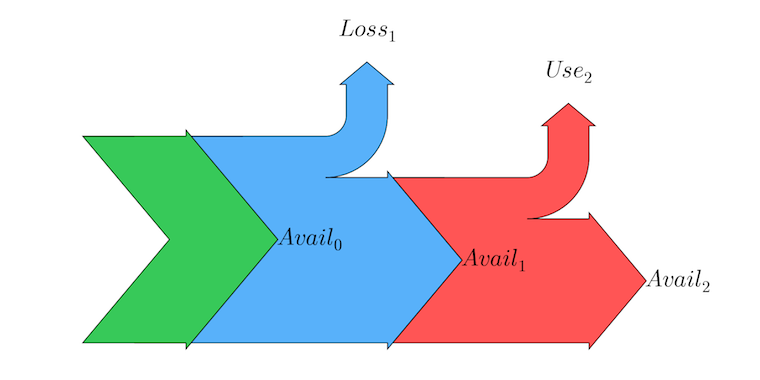# Modeling electricity losses in ecoinvent

## Input data and framework

Modeling electricity production, transformation, transmission and consumption is a bit tricky, as the data we have is a mix of relative and absolute values. In the world of life cycle inventory data collection, electricity is blessed with large amounts of data. However, when we look for data on the fraction of consumption that is high, medium, or low voltage, or when we want specific values for transformation or transmission losses, we have to use a few data points. In ecoinvent, these values come from the Swiss utilities, and are assumed to be the same worldwide. The data we have is the following:

• Total generation, total losses, and total consumption, per country or ecoinvent region. Note that electricity import and exports almost always happen at high voltages, so total generation is actually generation plus imports minus exports, but this doesn't change the loss calculations, so we ignore electricity trading.
• The fraction of total consumption which occurs at high (0.04), medium (0.32), and low voltage (0.64). In contrast with generation and consumption, these values are the same for all ecoinvent datasets.
• The fraction of the losses that occur at the the transformation and transmission steps (two different values) for high, medium, and low voltage. Again, these values are the same for all ecoinvent datasets:
Type Name Value
Transformation High voltage - high voltage 0.06
Transmission High voltage 0.33
Transformation High voltage - medium voltage 0.08
Transmission Medium voltage 0.05
Transformation Medium voltage - low voltage 0.24
Transmission Low voltage 0.24

It is very important to remember that these values are fractions of an absolute amount, not fractions of a functional unit, and can't be combined willy-nilly.

Let's visualize these values in a Sankey diagram, and show what values we know, and which values we have to calculate. For space reasons I don't show the whole electricity chain.It is worth repeating that we know the absolute amount of $Avail_{0}$, $Loss_{1}$, and $Use_{2}$, but we have to calculate $Avail_{1}$ and $Avail_{2}$. We also have to calculate the relative loss from $Loss_{1}$ per kilowatt-hour produced.

## Some math

We can write the general formula for available electricity at step n as:

\begin{equation*} Avail_{n} = Avail_{0} - \sum_{i=1}^{n} Loss_{i} - \sum_{i=1}^{n} Use_{i} \end{equation*}

Makes sense - the total available at any point is what we started with, minus losses and what was used. What about the relative amount of electricity lost at any particular point in the value chain? This is the number we need on ecoinvent - the fraction of one kilowatt hour gross availability which is lost. This loss coefficient (LC) is also quite easy to define:

\begin{equation*} LC_{i} = \frac{Loss_{i}}{Avail_{i - 1}} \end{equation*}

Remember, in both these equations, every input parameter is an absolute amount of electricity, not a fraction or percentage.

Let's look at a real world example: Lithuania, chosen because it has nice, small numbers. According to the input data used in ecoinvent, Lithuania has 11 TWh of generation, 10 TWh of consumption, and 1TWH of losses. Combined with our fixed fractions, we already know the following:We can also compare loss coefficients versus the values in the first table.

Type Name Value Loss coefficient
Transformation High voltage - high voltage 0.06 0.0055
Transmission High voltage 0.33 0.03
Transformation High voltage - medium voltage 0.08 0.0075
Transmission Medium voltage 0.05 0.0049
Transformation Medium voltage - low voltage 0.24 0.035
Transmission Low voltage 0.24 0.036

## Cumulative losses

Sometimes we want to know how much electricity is lost throughout the value chain; say, for example, you were curious how much electricity had to be generated to get 1 kilowatthour of medium voltage electricity supplied. In this case, we don't want the loss coefficient, but rather $1 - LC$, the amount of electricity provided after losses. We need to multiply this amount for each step where electricity is lost - it is just like interest from the bank, but in reverse. The formula for total fractional loss at step n is therefore:

\begin{equation*} TotalLossCoefficient_{n} = 1 - \prod_{i=1}^{n} \big( 1 - LC_{i} \big) \end{equation*}

The total loss coefficient for Lithuania for usage of medium voltage would include transformation and transmission losses for high and medium voltage, and would therefore be (with some rounding):

\begin{equation*} 1 - (1 - 0.0055) \cdot (1 - 0.03) \cdot (1 - 0.0075) \cdot (1 - 0.0049) = 0.0473 \end{equation*}

In words, the generation of 1 kilowatt hour of electricity would produce only 1 - 0.0473 kilowatt hours of medium voltage electricity at the busbar ("at the busbar" is what you say when you pretend to know something about electrical engineering).

Note that this value is not applicable to other countries, but depends on the country-specific ratio of total generation to total losses.